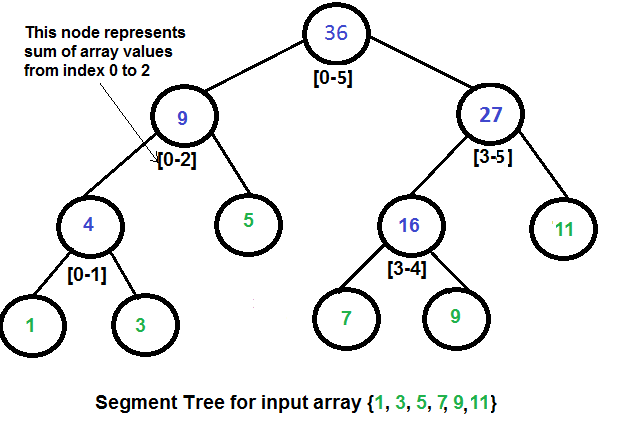## 线段树的概念

Tushar的讲解视频Segment Tree Range Minimum Query清晰明了。

## 线段树的例子

### Sum of given range

We have an array arr[0 . . . n-1]. We should be able to

1. Find the sum of elements from index l to r where 0 <= l <= r <= n-1
2. Change value of a specified element of the array to a new value x. We need to do arr[i] = x where 0 <= i <= n-1.

Representation of Segment trees

1. Leaf Nodes are the elements of the input array.
2. Each internal node represents some merging of the leaf nodes. The merging may be different for different problems. For this problem, merging is sum of leaves under a node.

An array representation of tree is used to represent Segment Trees. For each node at index i, the left child is at index `2*i+1`, right child at `2*i+2` and the parent is at`(i-1)/2`.Construction of Segment Tree from given array
We start with a segment arr[0 . . . n-1]. and every time we divide the current segment into two halves(if it has not yet become a segment of length 1), and then call the same procedure on both halves, and for each such segment, we store the sum in the corresponding node.
All levels of the constructed segment tree will be completely filled except the last level. Also, the tree will be a Full Binary Tree because we always divide segments in two halves at every level. Since the constructed tree is always a full binary tree with n leaves, there will be n-1 internal nodes. So total number of nodes will be 2*n – 1.

Height of the segment tree will be `logN`. Since the tree is represented using array and relation between parent and child indexes must be maintained, size of memory allocated for segment tree will be `2*pow(2, logN)-1`.
Query for Sum of given range
Once the tree is constructed, how to get the sum using the constructed segment tree. Following is the algorithm to get the sum of elements.

Update a value
Like tree construction and query operations, the update can also be done recursively. We are given an index which needs to be updated. Let diff be the value to be added. We start from root of the segment tree and add diff to all nodes which have given index in their range. If a node doesn’t have given index in its range, we don’t make any changes to that node.
Implementation
Following is the implementation of segment tree. The program implements construction of segment tree for any given array. It also implements query and update operations.

Output:

Time Complexity
Time Complexity for tree construction is O(n). There are total 2n-1 nodes, and value of every node is calculated only once in tree construction.

Time complexity to query is O(logN). To query a sum, we process at most four nodes at every level and number of levels is O(logN).

The time complexity of update is also O(logN). To update a leaf value, we process one node at every level and number of levels is O(logN).